# Graphing a System of Quadratic Inequalities: Examples & Process

Lesson Transcript
Instructor: Yuanxin (Amy) Yang Alcocer

Amy has a master's degree in secondary education and has been teaching math for over 9 years. Amy has worked with students at all levels from those with special needs to those that are gifted.

A system of quadratic inequalities is a collection of such functions, put into a set, or a 'whole'. Learn the process of how to graph a system of quadratic inequalities and view examples. Updated: 09/30/2021

First, what is a quadratic inequality? Well, the word quadratic tells us that we will have an x^2 term and that 2 will be our highest exponent. We might have an x term as well as a constant term, but these aren't required for quadratics. The inequality tells us that we are dealing with a function that may not be exactly equal. So we are dealing with greater than, less than, etc.

So we can define a quadratic inequality as a function of a quadratic that may not be exactly equal. We know that a quadratic equation looks like y = ax^2 + bx + c. Our quadratic inequality looks very similar except that we don't have an equal sign. Instead of an equal sign, we will have greater than, less than, greater than or equal to, or a less than or equal to symbol.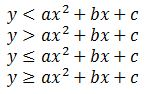I've written these in standard form, where a, b, and c stand for numbers. But remember that for a quadratic, the only term that must be there is the first x^2 term. What does this mean? It means that while b and c can be 0, essentially deleting those terms, a must be a number and cannot be 0. If we plug in random numbers, we can see how a quadratic inequality will look.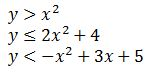We see that we can have one term, or two, or all three. But the one term that has to stay is the x^2 term.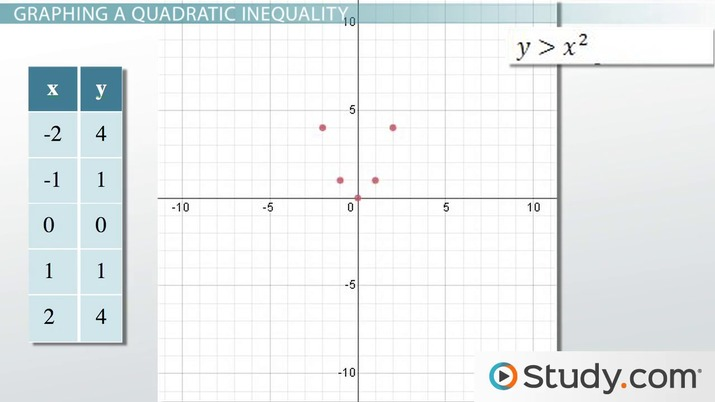An error occurred trying to load this video.

Try refreshing the page, or contact customer support.

Coming up next: How to Multiply and Divide Rational Expressions

### You're on a roll. Keep up the good work!

Replay
Your next lesson will play in 10 seconds
• 7:45 Lesson Summary
Save Save

Want to watch this again later?

Timeline
Autoplay
Autoplay
Speed Speed

While a quadratic inequality is just one of those functions, a system of quadratic inequalities is a collection of quadratic inequality functions considered as a set. What this means is that we will have more than one quadratic inequality, and when we consider these inequalities, we consider them as a whole. Any solution to one function must also be a solution to the other functions in the system.

When you have only one function, a solution only needs to satisfy that one function, but for a system, the solution must satisfy all the functions in the system. In math, we have a special notation for systems. We use a big curly bracket in front of the system like this: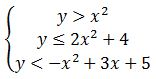A good way to see the solutions to a system of quadratic inequalities is to graph it. Graphing a system of quadratic inequalities is very similar to graphing a quadratic function by itself. The only difference is that we will be shading the area where the solutions are, and also the line we draw is determined by the inequality sign. Let's discuss these things by first graphing our first quadratic inequality: y > x^2.

As with other quadratics, we can either punch this function into a graphing calculator or make a table with points to plot. What I'm going to do is make a table with points. I'm going to choose the x values and calculate the y values using the x values. The x values I'm going to choose are -2, -1, 0, 1, and 2. To find each point, I plug in my x value wherever I see an x and then calculate to find my y. So, the first point is y = (-2)^2 = 4, which means my point is (-2, 4). I do this for each point to get a complete table.

x y
-2 4
-1 1
0 0
1 1
2 4

I plot these points on a graph to get my curve.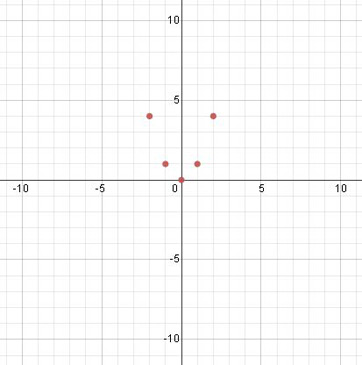To draw my curve, I need to look at the inequality sign I have. I see that I have a greater than symbol, so that means my line will be dashed. When graphing inequalities, if my symbol is greater than or less than, then my line is dashed. If the symbol is greater than or equal to or less than or equal to, then my line will be solid. I draw a dashed line for my curve since my symbol is greater than.

To unlock this lesson you must be a Study.com Member.

### Register to view this lesson

Are you a student or a teacher?